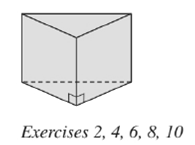Chapter 9.1, Problem 2EElementary Geometry For College St...

7th Edition
Alexander + 2 others
ISBN: 9781337614085

Solutions

Chapter
SectionElementary Geometry For College St...

7th Edition
Alexander + 2 others
ISBN: 9781337614085
Textbook Problem

Consider the solid shown. a ) Does it appear to be a prism? b ) It is right or oblique? c ) What type of base(s) does the solid have? d ) Name the type of solid. e ) what type of figure is each lateral face?To determine

a)

Whether the solid shown appear to be a prism.

Explanation

Calculation:

Given,

Since, in the above figure the two polygons, (triangle) at the top and another at the bottom appears to be congruent and also parallel, they can lie in two parallel planes...

To determine

b)

Whether the solid shown is right or oblique.

To determine

c)

The type of the base.

To determine

d)

The name of the type of the solid.

To determine

e)

The type of figure of each lateral face.

Still sussing out bartleby?

Check out a sample textbook solution.

See a sample solution

The Solution to Your Study Problems

Bartleby provides explanations to thousands of textbook problems written by our experts, many with advanced degrees!

Get Started

In Problems 19-44, factor completely. 23.

Mathematical Applications for the Management, Life, and Social Sciences

Domain Find the domain of the function. 17. f(x)=x+3x

Precalculus: Mathematics for Calculus (Standalone Book)

In Exercises 23-36, find the domain of the function. 26. g(x)=2x+1x1

Applied Calculus for the Managerial, Life, and Social Sciences: A Brief Approach

Express the function in the form f g h. 51. S(t) = sin2(cos t)

Single Variable Calculus: Early Transcendentals, Volume I

True or False: If a number p is in the interval of convergence of then so is the number .

Study Guide for Stewart's Single Variable Calculus: Early Transcendentals, 8th

Outline the major advantages and disadvantages of administering a survey by mail.

Research Methods for the Behavioral Sciences (MindTap Course List)

For

Study Guide for Stewart's Multivariable Calculus, 8th